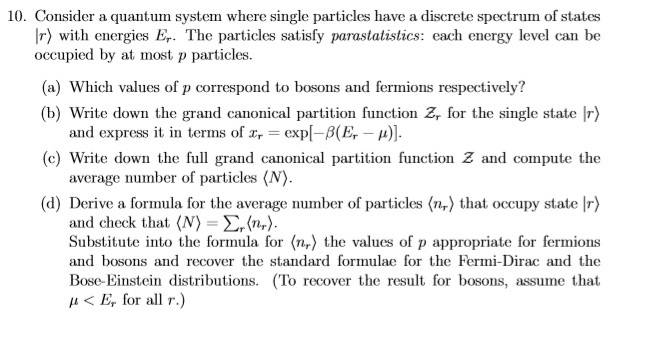# SM:Parastatistics, grand canonical ensemble function

• binbagsss
In summary, the conversation discusses a question regarding the grand canonical ensemble and the calculation of <N> and <n_r>. The individual steps for calculating these values are shown, but there are questions about convergence and the validity of the expressions, particularly for bosons. The conversation also mentions the recovery of the fermion distribution, which is found to be correct. The question is expected to verify the final, simplified expression for <N> and <n_r>.

## Homework Statement

Hi
I am looking at the question attached.Parts c and d, see below

## The Attempt at a Solution

First of all showing that ##<N> ## and ##<n_r>## agree

I have ##Z=\Pi_r z_r ##, where ##Z## here denotes the grand canonical ensemble.
So therefore we have ## \log(Z) = \sum\limits_r log (z) ## and since ##<N>=\frac{1}{\beta}\frac{\partial}{\partial \mu} \log Z ## the calculation seems pretty trivial unless there are some subtleties I am missing. The only subtlety I can think of however is the requirement of convergence such that the derivative can be taken inside the summation and then the derivative of the summation= sum of the derivative of each term, please see below.

So I have:
##z_r= \sum\limits_{n_r=0}^{n_r=p} x_r^{n_r } ##
so ## <n_r> =\frac{1}{\beta}\frac{\partial}{\partial \mu} \log ( \sum\limits_{n_r=0}^{n_r=p} x_r^{n_r } ) = \frac{1}{\beta} \frac{\sum\limits_{n_r=0}^{n_r=p} n_r \beta x_r^{n_r }}{\sum\limits_{n_r=0}^{n_r=p} x_r^{n_r } } ## (1)

And

##<N>= \frac{1}{\beta}\frac{\partial}{\partial \mu} \sum\limits_r \log ( \sum\limits_{n_r=0}^{n_r=p} x_r^{n_r } ) = \frac{1}{\beta} \sum\limits_r \frac{\partial}{\partial \mu} \log ( \sum\limits_{n_r=0}^{n_r=p} x_r^{n_r } ) = \frac{1}{\beta} \sum\limits_r \frac{\sum\limits_{n_r=0}^{n_r=p} n_r \beta x_r^{n_r }}{\sum\limits_{n_r=0}^{n_r=p} x_r^{n_r } } ## (2)

Where the 2nd equality has assumed convergence over the summation ## \sum\limits_r ##.

QUESTION 1- 2ND EQUALITY USING CONVERGENCE
So the question asks to use (1) and ## <N>= \sum\limits_r <n_r> ## to show that (2) is consistent with the latter. It is clear that the two agree. HOWEVER, I am unsure how to justify convergence over the summation of ##r##. Here ##r## is a single particle state, as far as I'm aware, (although most likely physically unlikely) this can be infinite, an ##n_r ## is the number of particles occupying a single ## r ## state. And so the summation over ##r## is infinite. If I ##E_r > \mu ## then this is an infinite summation of the logarithm of a summation which I know converges...but I don't think this really helps me out? (since i have ## \sum\limits_r \log (\sum\limits_{n_r} x_r^{n_r }) ## where ##x_r = \exp^{-\beta(E_r-\mu)}##).

QUESTION 2- UNABLE TO USE GENERAL EXPRESSION TO RECOVER BOSON DISTRUBUTION
so for fermions ##p=1## for bosons ##p=\infty##.
Looking at (1), the numerator multiplies ##n_r ## (dropped down from the exponentiial derivative) and so clearly when ##n_r=\infty ## this expression is dodgy/invalid- I am unsure what is wrong, however, with my above working.
The best I can seem to do is to recover the boson distribution from the expression at an earlier step, more generalised, which was:

## \frac{1}{\beta} \frac{\partial}{\partial \mu}( \log (\frac{1}{1-x_r})) ## it its easy to show that I attain ## \frac{1}{x_r^{-1}-1} ## as expected. (where I have use infinite summation formula for this and the assumption ## E_r > \mu ##
However I imagine the question wants you to be verifying your final, simplified expression. I am unsure what is wrong with (1)...

the fermion distribution recovery is ok:
## <n_r> = \frac{1}{\beta} \frac{\sum\limits_{n_r=0}^{n_r=1} n_r \beta x_r^{n_r }}{\sum\limits_{n_r=0}^{n_r=1} x_r^{n_r } } ##
this gives:
## \frac{1}{\beta}( 0 + \frac{\beta x_r}{1+x_r} )= \frac{1}{x_r^{-1}+1}## as expected.